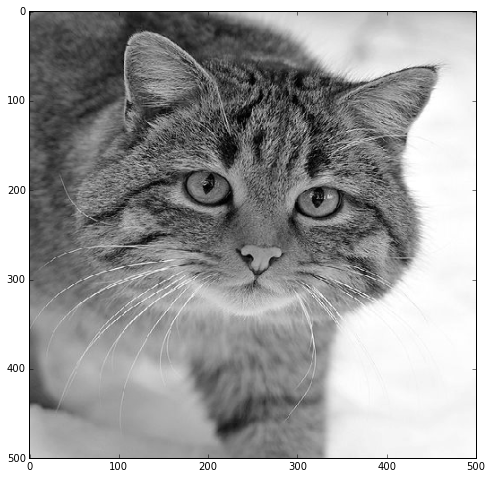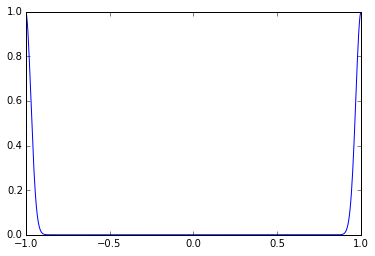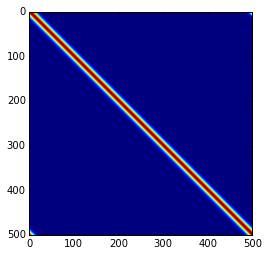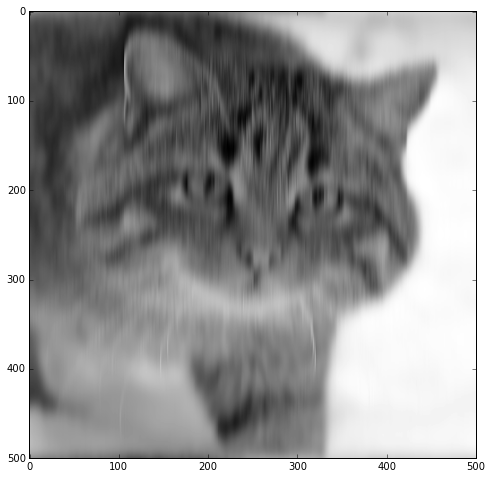# Matrices for image blurring

In :
import numpy as np
import matplotlib.pyplot as pt

In :
from PIL import Image

with Image.open("cat.jpeg").resize((500,500)) as img:
img = np.array(img).sum(axis=-1)

h, w = img.shape

/usr/lib/python3/dist-packages/PIL/Image.py:1531: ResourceWarning: unclosed file <_io.BufferedReader name='cat.jpeg'>


In :
pt.figure(figsize=(8,8))
pt.imshow(img, cmap="gray")

Out:
<matplotlib.image.AxesImage at 0x7ff8ecc44c50>Now make a Gaussian with as many pixels as the image is wide.

In :
x = np.linspace(-1, 1, w)
gaussian = np.exp(-500*x**2)
gaussian = np.roll(gaussian, -w//2)
pt.plot(x, gaussian)

Out:
[<matplotlib.lines.Line2D at 0x7ff8ecb5f588>]Now, fill a $w\times w$ matrix with shifted versions of this:

In :
A = np.zeros((w,w))
for i in range(w):
A[:, i] = np.roll(gaussian, i)


Here's a plot to show what just happened:

In :
pt.imshow(A)

Out:
<matplotlib.image.AxesImage at 0x7ff8ecac6898>Multiply the cat by this.

In :
blurrycat = A.dot(img)

In :
pt.figure(figsize=(8,8))
pt.imshow(blurrycat, cmap="gray")

Out:
<matplotlib.image.AxesImage at 0x7ff8eca9e828>In []: